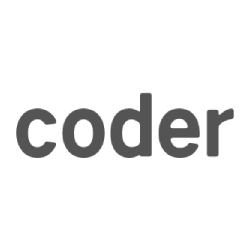# #POJ 3694 Network （无向图缩点 + LCA）

Network

 Time Limit: 5000MS Memory Limit: 65536K Total Submissions: 14538 Accepted: 5260

Description

A network administrator manages a large network. The network consists of N computers and M links between pairs of computers. Any pair of computers are connected directly or indirectly by successive links, so data can be transformed between any two computers. The administrator finds that some links are vital to the network, because failure of any one of them can cause that data can't be transformed between some computers. He call such a link a bridge. He is planning to add some new links one by one to eliminate all bridges.

You are to help the administrator by reporting the number of bridges in the network after each new link is added.

Input

The input consists of multiple test cases. Each test case starts with a line containing two integers N(1 ≤ N ≤ 100,000) and M(N - 1 ≤ M ≤ 200,000).

Each of the following M lines contains two integers A and B ( 1≤ A ≠ B ≤ N), which indicates a link between computer A and B. Computers are numbered from 1 to N. It is guaranteed that any two computers are connected in the initial network.

The next line contains a single integer Q ( 1 ≤ Q ≤ 1,000), which is the number of new links the administrator plans to add to the network one by one.

The i-th line of the following Q lines contains two integer A and B (1 ≤ A ≠ B ≤ N), which is the i-th added new link connecting computer A and B.

The last test case is followed by a line containing two zeros.

Output

For each test case, print a line containing the test case number( beginning with 1) and Q lines, the i-th of which contains a integer indicating the number of bridges in the network after the first i new links are added. Print a blank line after the output for each test case.

Sample Input

``````3 2
1 2
2 3
2
1 2
1 3
4 4
1 2
2 1
2 3
1 4
2
1 2
3 4
0 0
``````

Sample Output

``````Case 1:
1
0

Case 2:
2
0
``````

Accepted code

``````#include<iostream>
#include<algorithm>
#include<cstring>
#include<cstdio>
#include<queue>
#include<stack>
using namespace std;

#define sc scanf
#define ls rt << 1
#define rs ls | 1
#define Min(x, y) x = min(x, y)
#define Max(x, y) x = max(x, y)
#define ALL(x) (x).begin(),(x).end()
#define SZ(x) ((int)(x).size())
#define MEM(x, b) memset(x, b, sizeof(x))
#define lowbit(x) ((x) & (-x))
#define P2(x) ((x) * (x))

typedef long long ll;
const int MOD = 1e9 + 7;
const int MAXN = 5e5 + 100;
const int INF = 0x3f3f3f3f;
inline ll fpow(ll a, ll b){ ll r = 1, t = a; while (b){ if (b & 1)r = (r*t) % MOD; b >>= 1; t = (t*t) % MOD; }return r; }

struct Edge
{
int v, f, next;
}e[MAXN << 1], edge[MAXN << 1]; // 左为原图， 右为新图
int head[MAXN], pre[MAXN], n, m, cnt, tot, X;
int p[MAXN], dfn[MAXN], low[MAXN], q, ans;  // p为父亲
int suo[MAXN], sum[MAXN], dep[MAXN], scnt, stot;  // suo为缩点后的点， sum为边走过次数
stack <int> st;   // dep为深度
void init() {
MEM(head, -1); MEM(dfn, 0); MEM(low, 0);
MEM(pre, -1); MEM(dep, 0); MEM(suo, 0);
cnt = scnt = tot = stot = 0;
}
void add(int from, int to) {
e[cnt].v = to;
}
void Add(int from, int to) {
edge[++stot].v = to;
edge[stot].next = pre[from];
pre[from] = stot;
}
void tarjan(int x) {
dfn[x] = low[x] = ++tot;
st.push(x);
for (int i = head[x]; i != -1; i = e[i].next) {
int vi = e[i].v;
if (e[i].f) continue;
e[i].f = e[i ^ 1].f = 1;
if (!dfn[vi]) {
tarjan(vi);
low[x] = min(low[x], low[vi]);
}
else low[x] = min(low[x], dfn[vi]);
}
if (dfn[x] == low[x]) {
scnt++;
int k;
do {
k = st.top();
st.pop();
suo[k] = scnt;
} while (k != x);
}
}
void dfs(int x, int fa) {
dep[x] = dep[fa] + 1; p[x] = fa;
for (int i = pre[x]; i != -1; i = edge[i].next) {
int vi = edge[i].v;
if (vi != fa) dfs(vi, x);  // 更新深度和父亲
}
}
void LCA(int x, int y) {
if (dep[x] > dep[y]) swap(x, y);
while (dep[y] - 1 >= dep[x]) {
if (sum[y] == 1) ans--, sum[y]++;  // 先放到同一深度
y = p[y];
}
if (x == y) return;
while (x != y) {
if (sum[x] == 1) ans--, sum[x]++;  // 有必要的话一起往上跳
if (sum[y] == 1) ans--, sum[y]++;
x = p[x], y = p[y];
}
}

int main()
{
while (~sc("%d %d", &n, &m) && n + m) {
init();
for (int i = 0; i < m; i++) {
int ui, vi;
sc("%d %d", &ui, &vi);
}
for (int i = 1; i <= n; i++) {
if (!dfn[i]) tarjan(i);
}
for (int i = 1; i <= n; i++) {
sum[i] = 1;
for (int j = head[i]; j != -1; j = e[j].next) {
int ui = suo[i], vi = suo[e[j].v];
if (ui != vi) Add(ui, vi);
}
}
ans = scnt - 1; dfs(1, 1);
cin >> q;
cout << "Case " << ++X << ":" << endl;
while (q--) {
int ui, vi;
sc("%d %d", &ui, &vi);
LCA(suo[ui], suo[vi]);
cout << max(ans, 0) << endl;
}
cout << endl;
}
return 0;
}
``````

05-26

05-27

05-27

05-27

05-27

05-27

05-27

05-27

05-28

05-29

05-30

05-30

05-30

05-31

05-31

06-01